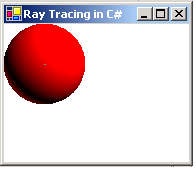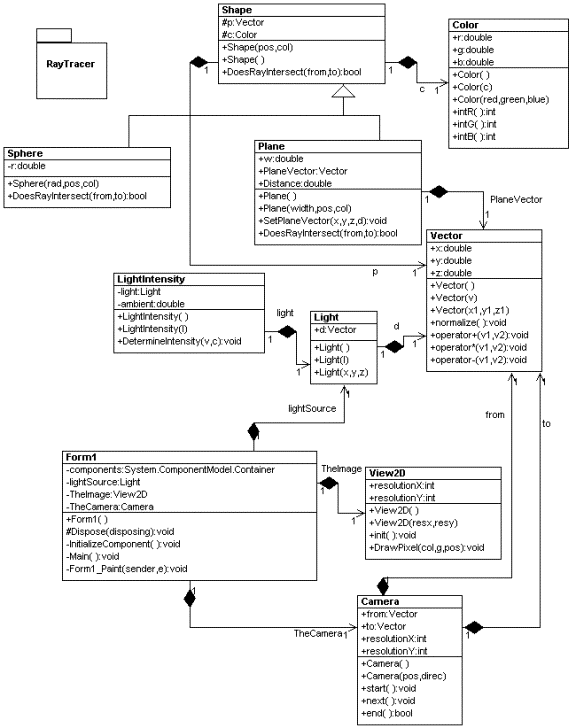In this article, I will show you how to implement ray tracing in C# and .NET.Figure 1 Ray Tracing a Sphere

I just saw the Japanese Animation movie Spirited Away and couldnt help admiring the combination of cool moving graphics, computer generated backgrounds, and integration of sound. This inspired me to revisit the world of 3-D computer graphics. One of the coolest techniques in generating 3-D objects is known as ray tracing. This technique utilizes how the behavior of a particle of light (a photon) with a solid or refractive object. We are only interested in the rays that reflect into the camera or view area near the eye. This view area forms the image from the different colors and intensities bouncing back from the solid object.

Sounds more like a science project than a computer program, but the ray tracing algorithm is pure mathematics (geometry and algebra).  The sphere is the simplest object to ray trace, because the sphere can be described in one simple formula, where other shapes such as a cube need to be described as the combination of several planes. The formula for the sphere is:

 (xs xc)2  + (ys yc)2 + (zs zc)2 rs2 = 0 where: xs is the xcoordinate of a point on the sphere xc is the x coordinate of the center of the sphere rs is the radius of the sphere

The majority of calculation in Ray Tracing is in finding a method to test for the intersection point of the object you are viewing.  By the way, we are not concerned with speed in this program.  If we were concerned with speed, we would use a watered-down version of ray tracing known as ray casting which is the technique used to form the graphics you see in Doom, Quake, Duke Nukem and other animation software that requires quick rendering.

The basic equation for a ray (or line)  in 3D space is shown below:

 R(t) = Ro  +  Rd * t    ( t > 0) where: Ro is the origin point of the ray at (xo, yo, zo) Rd is the direction of the ray at (xd, yd, zd) and is a unit vector (xd2  +   yd2  +   zd2  = 1) rs is the radius of the sphere

You can almost think of it as the formula of a line in 3d space where Ro is the y intercept and Rd is the slope.  Now lets look at a use case of the ray tracing algorithm

1. For each pixel on the surface of a 2-D screen that the eye or camera is viewing, determine the world coordinates of the pixel.
2. Cast a ray from the camera through the pixel.
3. Check for an intersection with a solid object (in this case a sphere.)
4. For the nearest intersection with the solid object, determine a shade corresponding to the distance of the ray to the object.

Lets look at the math in the algorithm and take a look at the Ray tracing formula broken up into its Cartesian coordinates:

 x(t) = xo  +  xd * t     (t > 0) y(t) = yo  +  yd * t    z(t) = zo  +  zd * t

If we now substitute the ray formula into the equation for a sphere we get:

 (xo  +  xd * t     xc)2  + (yo  +  yd * t     yc)2 + (zo  +  zd * t     zc)2 rs2 = 0

This formula can be solved for t by using the quadratic equation where:

 A = xd2  +   yd2  +   zd2   = 1 (because its a unit vector) B = 2 [xd(xo - xc) + yd(yo - yc) + zd(zo - zc) ] C = (xo - xc)2 + (yo -yc)2+ (zo - zc)2

So by the quadratic formula :

 tintersect =   -B  +/- Sqrt ( B2 4C)/2

The discriminant B2 4C must be greater than zero or there is no intersection (B2 4C < 0 produces an imaginary root).  Also we are only interested in the smallest root solution of  t that is greater than zero.  Even if the larger root of t is positive,  this root would be passing through the opposite side of the sphere, which is the side we cannot see, so we throw this root out.

Now we have everything we need to determine the point of intersection and then the Normal vector.  The normal vector is the vector perpendicular to the ray of intersection.  We need this to get our final pixel.

The point of intersection is the solution that we came up for t plugged back into the vector:

 (xi, yi,  zi,) = (xo + xd  * tintersect,  yo + yd  * tintersect,   zo + zd  * tintersect)

The normal vector at the point of intersection is determined from the formula below:

 (xn, yn,  zn,) =  (xi xc)/rs,  (yi yc)/rs,  (zi z c)/rs,

Lets go through the algebraic use case for calculating the pixels in the view again

1. Calculate the coefficients A, B and C from the quadratic solution using  the direction vector of the ray,  the ray origin point,  the center point of the sphere, and the radius of the sphere.
2. Use A, B, and C to calculate the point where the ray intersects with the sphere.
3. If the discriminant B2 4C  is less than 0,  the ray does not intersect.
4. If  one of the roots of the solution t = -B  +/- Sqrt (B2 4C)/2 has a root where t< 0,  then we throw out the root.
5. We want all solutions where t >= 0 but is the smaller of the two roots.
6. Use t to calculate the intersection point.
7. Use the intersection point to calculate the normal vector

Once we determine if the ray intersects with the object,  we need to determine the shading of the pixel based on the vector of a light source.  The shading of the pixel is determined by  the inner product of the light source vector with the normal vector ray intersecting the object  plus any ambient light.

 Shading  =   | Rn * La  +  ambient |

Now we have everything we need to create our ray-traced sphere in software.  Below is the UML design of our ray tracing C# program divided into the core objects Shape, Vector, Light, LightIntensity, Color, Camera, View2D.  The objects Sphere and Plane are objects for viewing that inherit from the Shape object and override the DoesRayIntersect method.  Light and LightIntensity classes are used to determine the shading of the object based on the formula above.  The View2D class is used to draw the pixel from the intersecting ray and the color determined from the Shading formula to the Form.  The Camera class is used to determine each sample ray being passed to the algorithm.  Most of the algorithm occurs in the overridden DoesRayIntersect method of the different shapes which not only determines if the ray sample intersects the object, but returns where it intersects if it does.Figure 2 UML Diagram of Ray Tracing Algorithm, reverse engineered using the WithClass UML Tool

The DoesRayIntersect method of the sphere used to determine the intersection of the ray with the sphere is shown below.  This method is called in the Form's Paint Event Handler for each pixel in the normalized camera grid to test and find the intersection of the ray from the camera to the sphere object and draws it if it exists.  Note how DoesRayIntersect steps through each step of the algorithm and checks t along the way to make sure its a valid ray intersecting with the sphere.

public override bool DoesRayIntersect(Vector Ro, Vector Rd, ref Vector v)
{
// Determine initial position (Ro - Rc) = (origin of ray - sphere center)
Vector vec = Ro - Rc;
// Use Rd and vec to determine the b component of the quadratic for
// the determinant by taking the dot product
double b = Rd * vec;
// calculate the c component of the quadratic from (Ro-Rc)^2 - (radius of sphere)
double
c = vec * vec - radius * radius;
// calculate the discriminant
double discriminant= b * b - c;
// if the discriminant< 0
// there is no intersection, return
if (discriminant< 0)
return false;
// calculate t for the ray
double dsquared = Math.Sqrt(discriminant);
double t = -b + dsquared ;
// if t is less than zero, the ray is coming from inside the sphere, skip it.
// We only want the smallest root, t, that is positive.
if(t < 0)
return false;
// t meets all the necessary conditions.
// calculate the intersecting vector by substituting t back in
Vector v1 = Rd * t + Ro;
v1 = v1 - Rc;
// normalize the vector (scale the vector such that x^2 + y^2 + z^2 = 1)
v1.normalize();
v = v1;
return true;
}

After the intersection is determined and the normal vector is computed, we can combine the vector with the lighting vector to get a color for the vector.  This is done with our LightIntensity object in the DetermineIntensity method shown below:

public void DetermineIntensity(Vector rayVector, ref Color aColor)
{
// take the dot product of the light source vector and the vector we just determined
// add the ambient value
// use the red color for shading
aColor.red = (lightSource.V * rayVector) + ambientLight;
if (aColor.red < 0)
aColor.red = -c.red;
aColor.green = 0.0;
aColor.blue = 0.0;
}

We can also draw the 3D vector on a 2D surface using the x and y coordinates of the ray with the help of  the DrawPixel method in the View2D class.  We'll use the resolution of the surface to scale the vector up so we can see it on the form.  Also note that we add one to the position to make sure its not sitting in the negative plane of the form.  A pen is created using  the color we just came up with in our LightIntensity Object.

public void DrawPixel(Color color, Graphics g, Vector position)
{
// scale up the vector x and y coordinates so we can see them on the form.
// Also translate them into the positive plane.
int x = (int) ((position.x + 1.0) * (resolutionX/5));
int y = (int)((position.y + 1.0) * (resolutionY/5));
// Draw a pixel according to the color that we determined from our LightIntensity class. Convert our Color class
// to the internal .NET color class using the Argb function
g.DrawLine(new Pen(System.Drawing.Color.FromArgb(color.GetRed(), (int)color.GetGreen(),
(
int)color.GetBlue()), 1), (int)x, (int)y, (int)x+1, (int)y);
}

Drawing a Plane

Although we didn't talk about them in this article, the code also contains ray tracing for planes as well.  If you want to examine ray tracing of planes, you can try uncommenting the code in the Form for creating a plane and comment out the code for drawing a sphere.   The algorithm for drawing a plane is slightly different, although we derive t through the same algebraic method we did for the sphere.  Some of the references below will give you a good idea on how  a plane is ray-traced.

References on the Web

This article was developed referencing the information from the following links:

Conclusion

Ray tracing is a very powerful demonstration of what a computer is capable of graphically purely through mathematics. With other techniques such as refraction and texture mapping you can produce some pretty awesome spectacles on your computer screen.  Have fun tracing your steps in .NET!